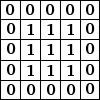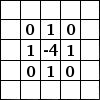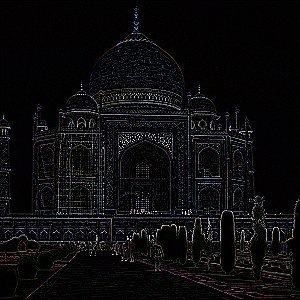[TOC]

CNNs were responsible for major breakthroughs in Image Classification and are the core of most Computer Vision systems today, from Facebook’s automated photo tagging to self-driving cars.

## What is Convolution

below is a Convolution with 3x3 Filter.as you can see, a convolution is like a sliding window function applied to a matrix. the sliding window is called a kernel, filter or feature detector.

Here we use a 3×3 filter, multiply its values element-wise with the original matrix, then sum them up. To get the full convolution we do this for each element by sliding the filter over the whole matrix.

EXAMPLES:

• Averaging each pixel with its neighboring values can blur an image.• taking the difference bewteen a pixel and its neighbours detect eges## What are CNNs

CNNs are basically just several layers of convolutions with nonlinear activation functions like ReLU or tanh applied to the results.

In a traditional feedforward neural network we connect each input neuron to each output neuron in the next layer. That’s also called a fully connected layer, or affine layer.

In CNNs we don’t do that. Instead, we use convolutions over the input layer to compute the output. This results in local connections, where each region of the input is connected to a neuron in the output. Each layer applies different filters, typically hundreds or thousands like the ones showed above, and combines their results. There’s also something something called pooling (subsampling) layers,During the training phase, a CNN automatically learns the values of its filters based on the task you want to perform.

For example, in Image Classification a CNN may learn to detect edges from raw pixels in the first layer, then use the edges to detect simple shapes in the second layer, and then use these shapes to deter higher-level features, such as facial shapes in higher layers. The last layer is then a classifier that uses these high-level features.